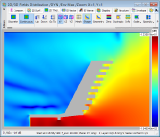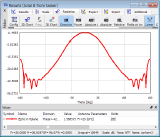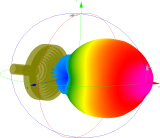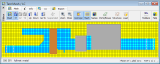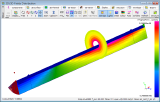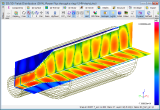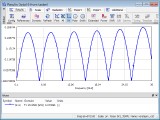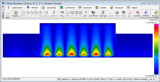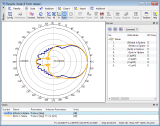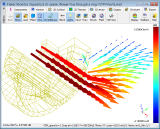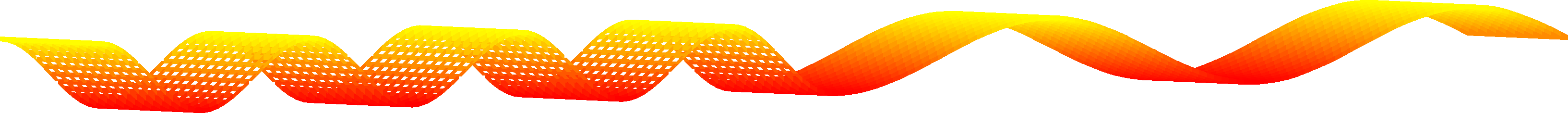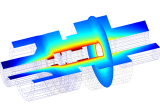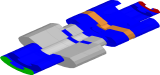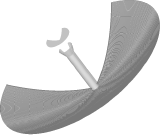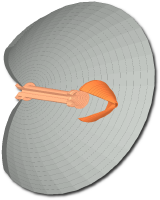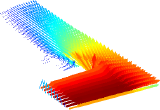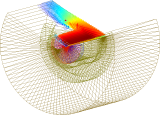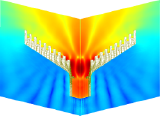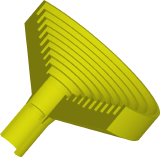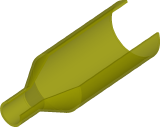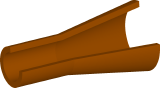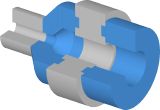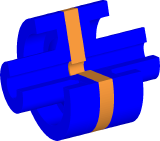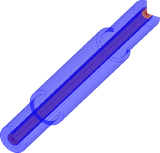QuickWave V2D
Vector 2D (V2D) solver for axisymmetrical structures (BOR - Bodies of Revolution).

QuickWave V2D is a unique on the market and ultra fast Vector 2D (QW-V2D) electromagnetic solver is applicable to the analysis of axisymmetrical devices (which are also called Bodies Of Revolution) as large as 2100 wavelengths, including antennas (horns, rods, biconical), circular waveguide discontinuities, and resonators. It is based on the Maxwell equations re-expressed in cylindrical coordinates. Definition of a 2D long-section of the structure allows for hundreds times faster simulation than brute force 3D analysis.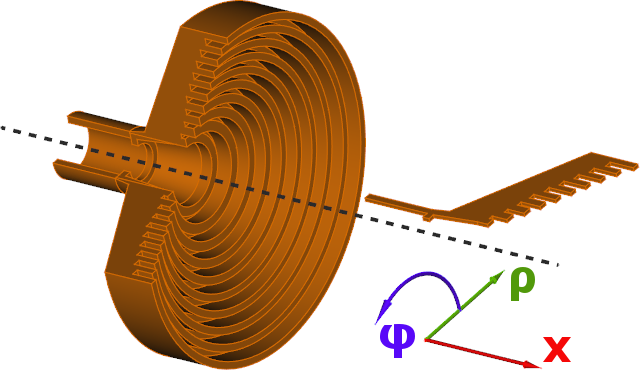It has been proven  that the structures, which maintain axial symmetry of boundary conditions, belong the class of vector two-dimensional (V2D) problems. The total electromagnetic field in such structures can be decomposed into a series of orthogonal modes, of different angular field dependence of the cos(nPhi) or sin(nPhi) type, where Phi is angular variable of the cylindrical coordinate system and n=0,1,2... Each n-mode is analysed separately in QW-V2D. Under such assumptions the numerical analysis can be conducted in two space dimensions, over one half of the long-section of the structure, with n predefined as a parameter. Let us note that the n-mode defined above should not be confused with one mode of a circular waveguide. For example, one QW-V2D analysis with n=1 takes into account a composition of all circular waveguide modes TE1k and TM1m where k and m are arbitrary natural numbers.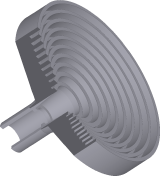QuickWave V2D utilises the conformal FDTD method in a vector two-dimensional formulation, expressed in cylindrical coordinates.
QuickWave V2D can be applied to a variety of microwave and millimetre-wave problems including:
calculations of radiation patterns, gain, radiation efficiency, and return loss of axisymmetrical antennas of various types (horn, rod, biconical), rigorously taking into account irregular geometry, complicated corrugations, and inhomogeneous filling
calculations of radiation patterns and radiation resistance of small dipole or loop radiators, located at the axis of the cylindrical coordinate system
accurate S-parameter calculations of circular waveguide discontinuities, also in cases involving strong dispersion and multimodal propagation
determination of eigenfrequencies, Q-factors, and pure modal field patterns for shielded and open inhomogeneous axisymmetrical resonators, also in cases involving closely-spaced modes or whispering gallery modes
calculation of heating patterns and specific absorption rate in axisymmetrical bodies
QuickWave V2D is the basic software in designing of two-reflectors Cassegrain antennas
used in telescopes as well as communication antennas.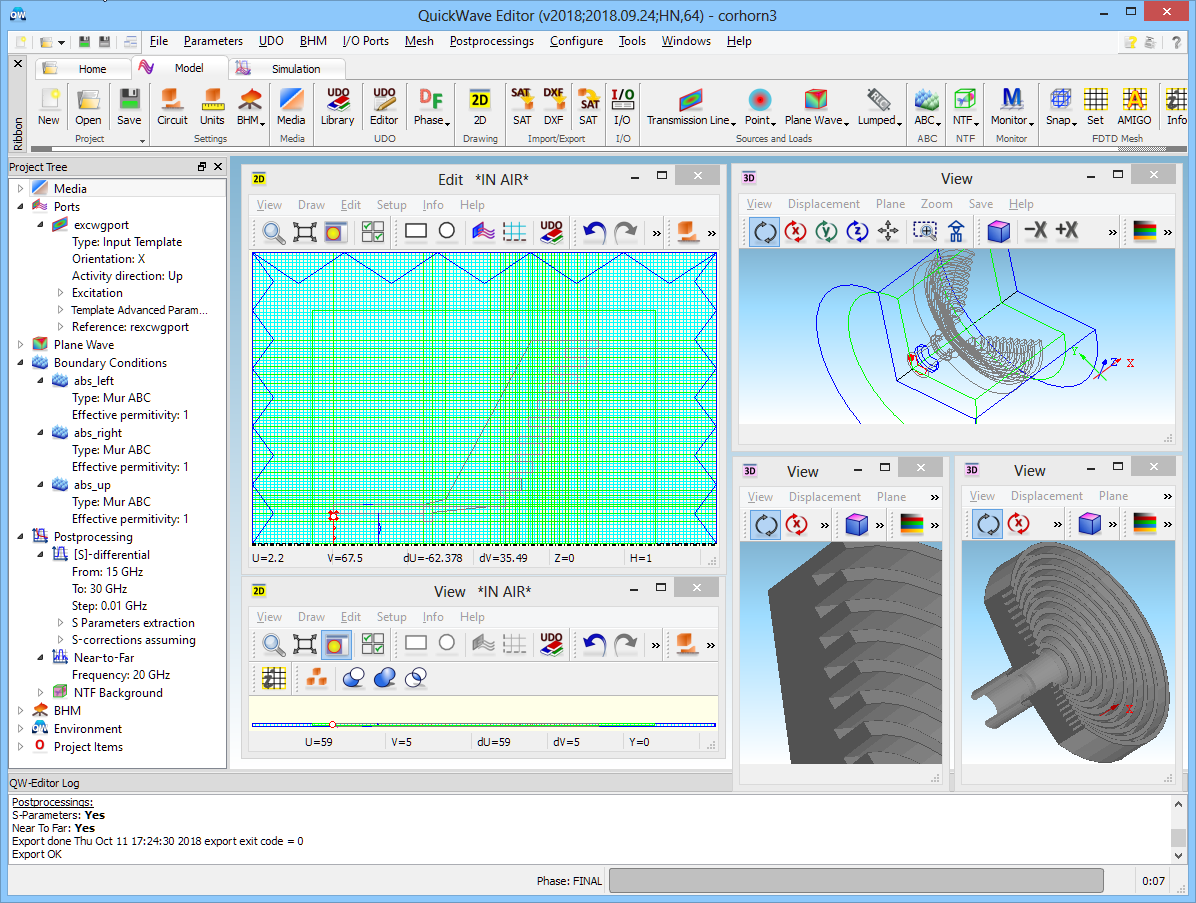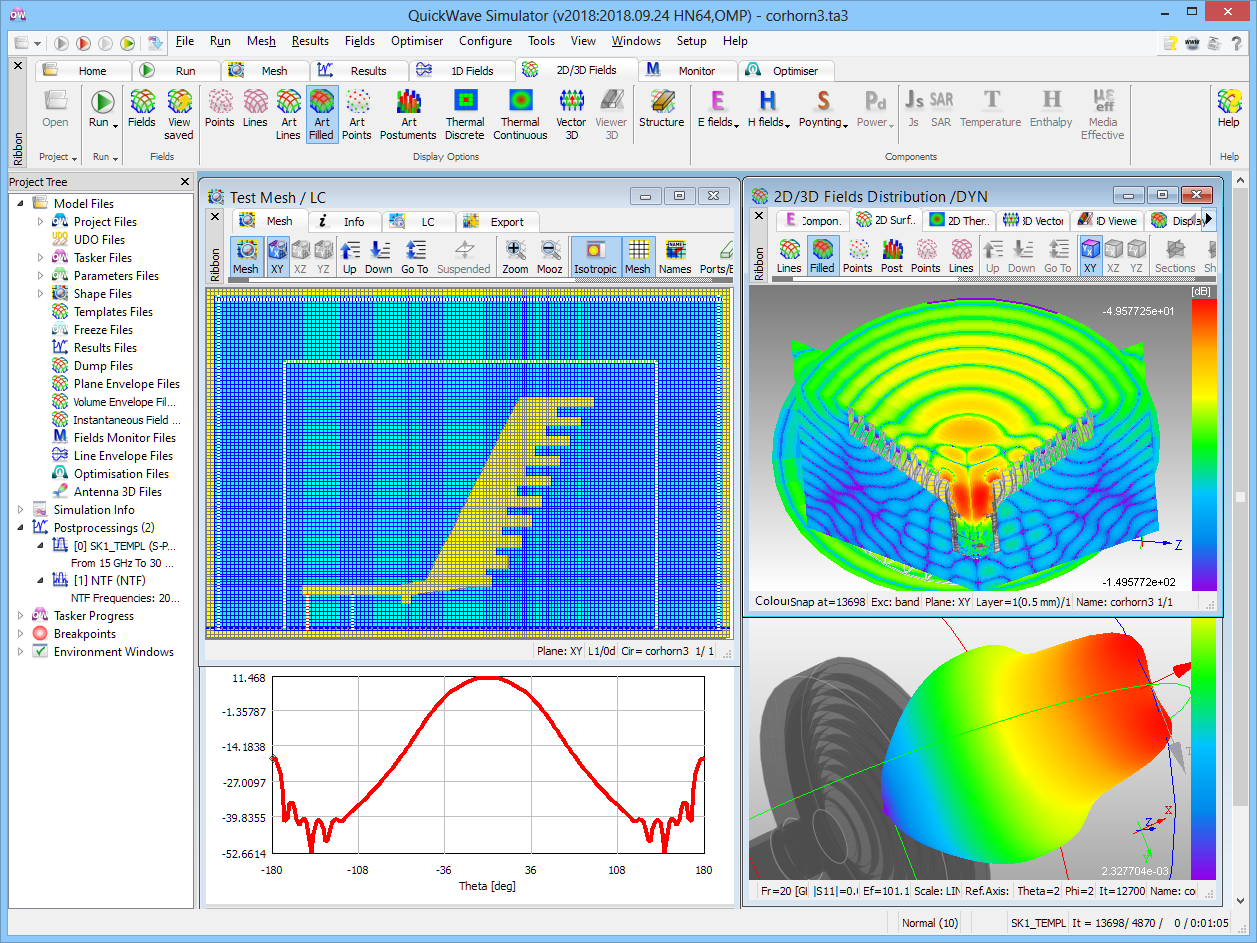See also a "Industrial Design of Axisymmetrical Devices Using a Customized FDTD Solver from RF to Optical Frequency Bands" paper reviewing fascinating applications of the QW-V2D software that was published in the IEEE Microwave Magazine, vol. 9, No.6.